# Irrigatable Area As Limited By Water Supply

This calculates the land area that can be irrigated with a given flow of water. The minimum system capacity (supply) is the available water from the supply. The water needs is the peak crop water need during a specific time period. Go here, or here for estimates for Washington. The available hours of operation per day is measured as the available hours for irrigation on a worst case day. The system efficiency is based on the irrigation efficiency and distribution uniformity. Learn more about the units used on this page.

Minimum System Capacity (Supply):
Operation Hours Per Day:
System Efficiency:
Irrigatable Area:

## The Equation

This calulator uses this formula to determine the Irrigatable area.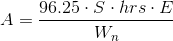Where: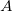= Irrigatable Area (sq. ft)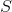= Minimum system capacity, or supply (gpm)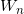= Water needs (in/day)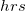= Operation hours per day (hrs)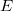= System Efficiency (as a decimal)

WSU Prosser - IAREC, 24106 N Bunn Rd, Prosser WA 99350-8694, 509-786-2226. Contact Us